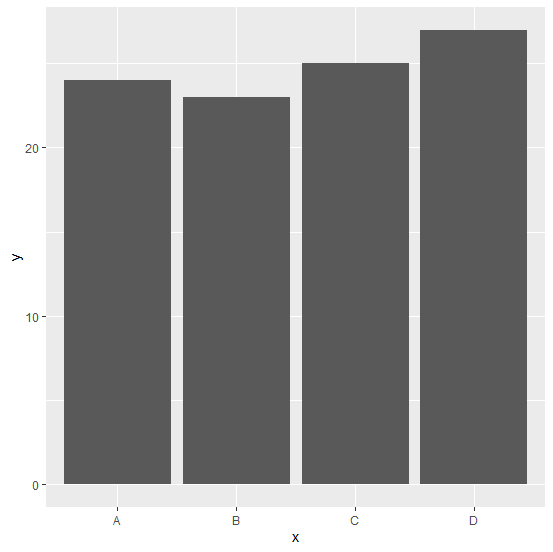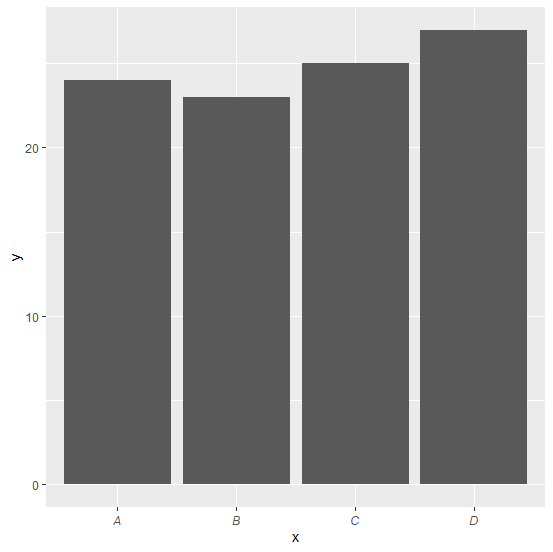# How to convert the X-axis label in a bar plot to italic using ggplot2 in R?

Obviously, the default font of axes-labels is not italic in R just like any other statistical analysis tool but we can make it using ggplot2. For this purpose, we can use theme function of ggplot2 package where we have an option to change the font of the axis labels using axis.text.x argument.

## Example

Consider the below data frame:

Live Demo

> x<-c("A","B","C","D")
> y<-c(24,23,25,27)
> df<-data.frame(x,y)
> df

## Output

  x  y
1 A 24
2 B 23
3 C 25
4 D 27

## Example

> library(ggplot2)
> ggplot(df,aes(x,y))+geom_bar(stat="identity")

## Output:Creating bar plot with italic X-axis labels:

## Example

> ggplot(df,aes(x,y))+geom_bar(stat="identity")+theme(axis.text.x=element_text(face=c("italic","italic","italic","italic")))
Warning message:
Vectorized input to element_text() is not officially supported.
Results may be unexpected or may change in future versions of ggplot2.

This warning message does not affect the plot but it helps us to understand that maybe the future versions of ggplot2 will not support vectorized input inside element_text.

## Output: